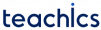A doubly linked list or two way linked list is a type of linked list that contains a pointer to the next node as well as the previous node in the sequence.

That is, each node in a doubly-linked list consists of:

• `data` – Data Item.
• `prev` – Address of previous node.
• `next` – Address of next node.

### Representation of Doubly Linked List

In C, we can represent a doubly linked list node using structures as:

```struct node
{
struct node *prev;
int data;
struct node *next;
};```

Each struct node contains a data item, a pointer to the previous struct node, and a pointer to the next struct node.

The following example creates a basic Linked List with three items and displays its elements.

### Example: Creating a Doubly Linked List

```#include <stdio.h>
#include <stdlib.h>

struct node
{
int data;
struct node *next;
struct node *prev;
};

int main()
{
// Create and initialize nodes
struct node *one = NULL;
struct node *two = NULL;
struct node *three = NULL;
struct node *current = NULL;

// Allocate memory for the nodes
one = (struct node*) malloc(sizeof(struct node));
two = (struct node*) malloc(sizeof(struct node));
three = (struct node*) malloc(sizeof(struct node));

// Assign data to nodes
one->data = 1;
two->data = 2;
three->data = 3;

// Connect first node
// with the second node
one->next = two;

// Connect second node
// with the third node
two->next = three;

// make next pointer of
//third node to NULL
//indicates last node
three->next = NULL;

//connect previous nodes
one->prev = NULL;
two->prev = one;
three->prev = two;

// print the linked list values forward
while(current != NULL)
{
printf("%d ", current->data);
current = current->next;
}

return 0;
}```

##### Output

`1 2 3`